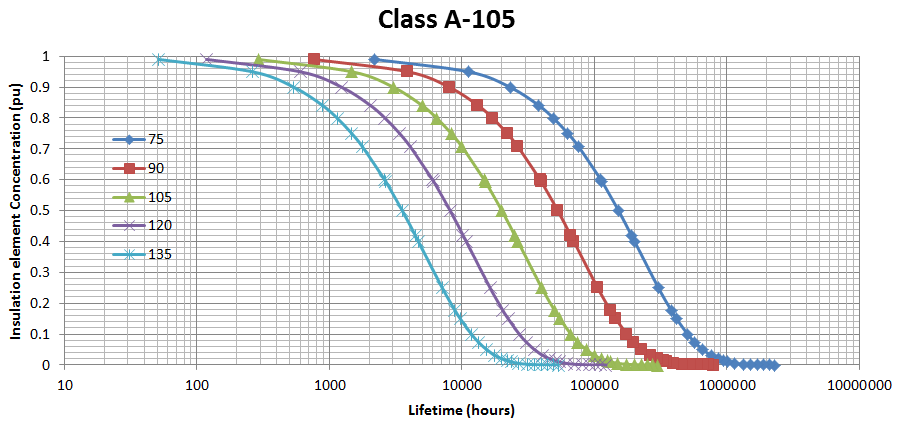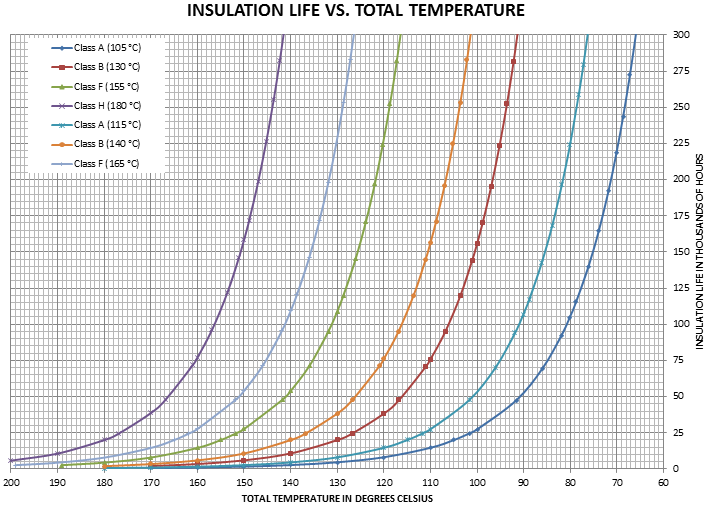###### Motor Insulation ClassesOne important reason for motor failure is the overheating condition. The current flowing in the motor originates a temperature rise which in turns degrades the insulation system as per​​ the Arrhenius equation for chemical reaction: The temperature rise in the windings affects the thermal insulation life expectancy.

The insulation thermal life is governed by a reaction rate equation of the first order.

dCdt=-kC

[C] is the insulation element concentration present in the chemical reaction (percent or per unit),​​ k​​ is the rate constant (sec-1). Integration of the above equation gives :

lnC= -kt+lnC0

[C]​​ 0​​ is the initial insulation element concentration at time​​ t=0.

The rate coefficient k is given by the Arrhenius​​ equation and is temperature dependent.

k=Ae-E/RT

A is the frequency constant (sec-1), E is the activation energy (kJ/mol), T is the temperature (°K) and R is the universal gas constant (8.314x10-3​​ kJ/mol/°K).

The parameters A and E are considered constants​​ for each insulation system.

The lifetime of the insulation system is usually defined as the time when the actual concentration reaches half of the initial concentration. That is​​ [C]​​ ½=0.5[C]​​ 0.

The insulation system temperature rating is obtained by test:​​ a sample of the insulation reacts at a fixed temperature. This is repeated for different temperatures and the temperature rating is established for a given lifetime (usually between 20 and 25 thousand hours). For example, the figure below shows five curves for a Class A insulation system. As said above, the insulation lifetime arrives when half of the concentration remains (0.5 pu in the graph). The temperature at which the 0.5 concentration is closest to the lifetime (that is 20 thousand hours) is 105°C.NEMA specifies letter designations for motor thermal insulation systems based on thermal endurance for temperature rating purposes. Thermal ratings are 40°C ambient temperature and the motor running continuously at service factor load.

The insulation life expectancy of a motor is around 20 to 25 thousand hours for the motor thermal system under the maximum total thermal ratings. The table below shows the allowable temperature rise.

NEMA Insulation Temperature Ratings

 NEMA INSULATIONCLASS Motor Service ​​ Factor Ambient Temperature (°C) Temperature Rise at service factor load (°C) HotspotTemperature(°C) Total Temperature(°C) A 1.0 40 60 5 105 1.15 40 70 115 B 1.0 40 80 10 130 1.15 40 90 140 F 1.0 40 105 10 155 1.15 40 115 165 H 1.0 40 125 15 180 1.15 40 … …

Thermal insulation life expectancy is not affected if temperatures are as shown in table above. Typical life expectancy versus temperature curves for NEMA Insulation Classes are shown in the figure below. This curve is given as a​​ reference and uses 20 thousand hours lifetime.

Each curve is identified by the NEMA insulation class and the allowable total temperature that is the first and the last columns shown in the table above.

The figure shows the insulation life for a continuous​​ total temperature. For example, the Class A (105°C) gives 20 thousand hours. If the actual total temperature is 90°C, the insulation life will be 52 thousand hours.The motor current (I) can be expressed as a per unit value of the Full Load Amps (FLA). The temperature rise is proportional to the motor square current (I2).

The table below shows the temperature rise for each insulation class following the motor current.

 I (pu) I2​​ (pu) Class A (105°C)Temp Rise (°C) Class A (115°C)Temp Rise (°C) Class B (130°C) Temp Rise (°C) Class B (140°C) Temp Rise (°C) Class F (155°C) Temp Rise (°C) Class F (165°C) Temp Rise (°C) Class H (180°C) Temp Rise (°C) 0 0 0 0 0 0 0 0 0 0.5 0.25 15 13.2 20 17.0 26.3 21.7 31.3 0.6 0.36 21.6 19.1 28.8 24.5 37.8 31.3 45.0 0.8 0.64 38.4 33.9 51.2 43.6 67.2 55.7 80.0 0.9 0.81 48.6 42.9 64.8 55.1 85.1 70.4 101.3 1 1 60 52.9 80 68.1 105 87.0 125 1.15 1.3225 79.35 70 105.8 90 138.9 115 165.3

This table can be used to​​ estimate the actual insulation lifetime of a motor given its actual loading.

Example :

What is the estimated motor insulation lifetime of a NEMA Class B (130°C) motor running at 0.9 times its​​ FLA?

The estimated temperature rise for this motor is 64.8 °C (as shown in the table above). The total temperature including ambient (40°C) and hotspot (10°C) temperatures is 114.8 °C. From the insulation life vs. total temperature curve the actual estimated motor insulation lifetime is 54​​ thousand hours.​​

Ernesto​​ Hidalgo-Tupia, Eng., M. A. Sc.​​
Electrical EngineerDownload PDF